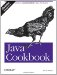# Recipe 10.5 Scanning Input with the 1.5 Scanner Class

#### Problem

You have JDK 1.5 and you want the ease of use that the java.util.Scanner class brings to simple reading tasks.

#### Solution

Use Scanner's next( ) methods for reading.

#### Discussion

The Scanner class lets you read an input source by tokens, somewhat analogous to the StreamTokenizer described in Recipe 10.4. The Scanner is more flexible in some ways it lets you break tokens based on spaces or regular expressions but less in others you need to know the kind of token you are reading. This class bears some resemblance to the C-language scanf( ) function, but in the Scanner you specify the input token types by calling methods like nextInt( ), nextDouble( ), and so on. Here is a simple example of scanning:

`// From ScannerTest.java String sampleDate = "25 Dec 1988";   Scanner sDate = Scanner.create(sampleDate); int dom = sDate.nextInt( ); String mon = sDate.next( ); int year = sDate.nextInt( );`

The Scanner recognizes Java's eight built-in types, as well as BigInteger and BigDecimal. It can also return input tokens as Strings or by matching regular expressions (see Chapter 4). Table 10-3 lists the "next" methods and corresponding "has" methods; the "has" method returns true if the corresponding "next" method would succeed. There is no nextString( ) method; just use next( ) to get the next token as a String.

##### Table 10-3. Scanner methods

Returned type

"has" method

"next" method

Comment

String

hasNext( )

next( )

The next complete token from this scanner

String

hasNext(Pattern)

next(Pattern)

The next string that matches the given regular expression (regex)

String

hasNext(String)

next(String)

The next token that matches the regex pattern constructed from the specified string

BigDecimal

hasNextBigDecimal( )

nextBigDecimal( )

The next token of the input as a BigDecimal

BigInteger

hasNextBigInteger( )

nextBigInteger( )

The next token of the input as a BigInteger

boolean

hasNextBoolean( )

nextBoolean( )

The next token of the input as a boolean

byte

hasNextByte( )

nextByte( )

The next token of the input as a byte

double

hasNextDouble( )

nextDouble( )

The next token of the input as a double

float

hasNextFloat( )

nextFloat( )

The next token of the input as a float

int

hasNextInt( )

nextInt( )

The next token of the input as an int

String

N/A

nextLine( )

Reads up to the end-of-line, including the line ending

long

hasNextLong( )

nextLong( )

The next token of the input as a long

short

hasNextShort( )

nextShort( )

The next token of the input as a short

The Scanner class does not provide any public constructors; you must call the static create( ) method with an input source, which can be a File object (Chapter 11), an InputStream, a String, or Readable (new in 1.5, Readable is an interface that Reader and all its subclasses implement).

One way of using the Scanner is based on the Iterator pattern, using while (scanner.hasNext( )) to control the iteration. Example 10-4 shows the simple calculator from Recipe 10.4 rewritten to use the Scanner class.

 If this were code in a maintained project, I might factor out some of the common code among these two calculators, as well as the one in Recipe 5.19, and divide the code better using interfaces. However, this would detract from the simplicity of self-contained examples.

##### Example 10-4. Simple calculator using java.util.Scanner
`import java.io.*; import java.util.Scanner; import java.util.Stack; /**  * SimpleCalc -- simple calculator using 1.5 java.util.Scanner  * @version    \$Id: ch10.xml,v 1.5 2004/05/04 20:12:12 ian Exp \$  */ public class SimpleCalcScanner {     /** The Scanner */     protected  Scanner scan;     /** The output */     protected PrintWriter out = new PrintWriter(System.out);     /** The variable name (not used in this version) */     protected String variable;     /** The operand stack */     protected Stack s = new Stack( );     /* Driver - main program */     public static void main(String[] av) throws IOException {         if (av.length == 0)             new SimpleCalcScanner(                 new InputStreamReader(System.in)).doCalc( );         else              for (int i=0; i<av.length; i++)                 new SimpleCalcScanner(av[i]).doCalc( );     }     /** Construct a SimpleCalcScanner by name */     public SimpleCalcScanner(String fileName) throws IOException {         this(new FileReader(fileName));     }     /** Construct a SimpleCalcScanner from an open Reader */     public SimpleCalcScanner(Reader rdr) throws IOException {         scan = new Scanner(rdr);         // Control the input character set:         scan.slashSlashComments(true);    // treat "//" as comments         scan.ordinaryChar('-');        // used for subtraction         scan.ordinaryChar('/');    // used for division     }     /** Construct a SimpleCalcScanner from a Reader and a PrintWriter */     public SimpleCalcScanner(Reader rdr, PrintWriter pw) throws IOException {         this(rdr);         setWriter(pw);     }     /** Change the output to go to a new PrintWriter */     public void setWriter(PrintWriter pw) {         out = pw;     }     protected void doCalc( ) throws IOException {         int iType;         double tmp;         while (scan.hasNext( )) {             if (scan.hasNextDouble( )) {                 push(scan.nextDouble( ));             } else {                 String token = scan.next( ).toString( );                 if (token.equals("+")) {                     // Found + operator, perform it immediately.                     push(pop( ) + pop( ));                 } else if (token.equals("-")) {                     // Found - operator, perform it (order matters).                     tmp = pop( );                     push(pop( ) - tmp);                 } else if (token.equals("*")) {                     // Multiply is commutative                     push(pop( ) * pop( ));                 } else if (token.equals("/")) {                     // Handle division carefully: order matters!                     tmp = pop( );                     push(pop( ) / tmp);                 } else if (token.equals("=")) {                     out.println(peek( ));                 } else {                     out.println("What's this? " + token);                 }             }         }     }     void push(double val) {         s.push(new Double(val));     }     double pop( ) {         return ((Double)s.pop( )).doubleValue( );     }     double peek( ) {         return ((Double)s.peek( )).doubleValue( );     }     void clearStack( ) {         s.removeAllElements( );     } }`Java Cookbook, Second Edition
ISBN: 0596007019
EAN: 2147483647
Year: 2003
Pages: 409
Authors: Ian F Darwin

Similar book on Amazon